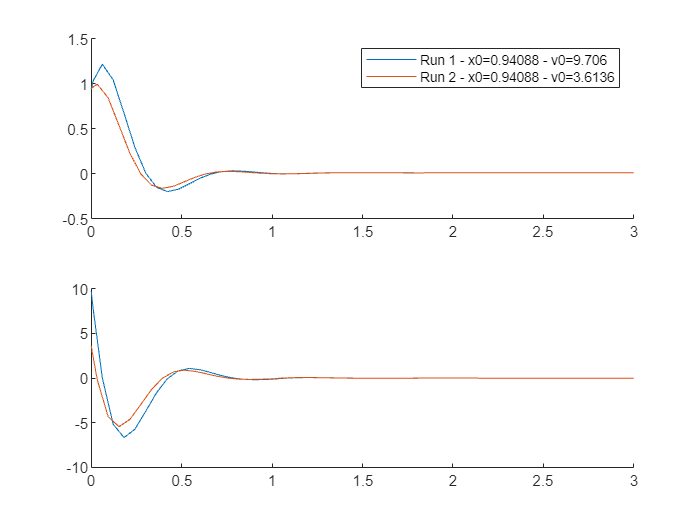# Function Handles and Post-Simulation Functions

Until recently, I always had trouble when dealing with MATLAB function handles. I cannot really explain why, there was just something I could not wrap my head around.
To help a user, I finally took the time to demystify that in my head and decided to share what I learned with you today.
In Simulink, the use cases requiring function handles that I run the most frequently into are:
In today's post, I will use a post-simulation callback for sim as an example.

## The Example

For this example, I will use this simple model where I output signals x and v using root-level Outport blocks: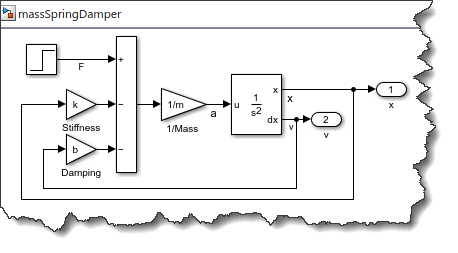I can simulate this model using a SimulationInput object:
mdl = 'massSpringDamper';
out = sim(in);
The SimulationOutput object contains the two logged signals
out.yout
ans =
Simulink.SimulationData.Dataset 'yout' with 2 elements Name BlockPath ____ __________________ 1 [1x1 Signal] x massSpringDamper/x 2 [1x1 Signal] v massSpringDamper/v - Use braces { } to access, modify, or add elements using index.

## A Simple Post Simulation Callback

In its simplest form, configuring a post-simulation callback is straightforward. Here is a screenshot from the documentation: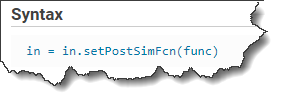The input func is a handle to a function that receives the original SimulationOutput object as input, and returns a structure with the fields to be included in a new SimulationOutput object. For example, I define this function:
function newout = myPostSim(out)
mySignal = out.yout.get('x').Values;
newout.mean = mean(mySignal);
newout.max = max(mySignal);
newout.min = min(mySignal);
end
Then to specify that this function needs to execute after the simulation, I pass to setPostSimFcn a handle to myPostSim using the "@" character:
mdl = 'massSpringDamper';
in = in.setPostSimFcn(@myPostSim);
out = sim(in)
out =
One thing to note, the SimulationOutput object returned in this case does not contain the original signal yout, it only contains the new fields created in the post-simulation function, along with the SimulationMedata and the ErrorMessage fields. This is especially useful if your simulation is producing a lot of data, but you are only interested in post-processed statistics, like in this case the mean, min and max.
If you want to keep the original data, you can simply re-assign it to the output. For the above example, this looks like:
function newout = myPostSim2(out)
newOut = out;
mySignal = out.yout.get('x').Values;
newout.mean = mean(mySignal);
newout.max = max(mySignal);
newout.min = min(mySignal);
end
When simulating, the SimulationOutput object contains the original yout and the newly computed values
mdl = 'massSpringDamper';
in = in.setPostSimFcn(@myPostSim2);
out = sim(in)
out =

When scrolling through the documentation for setPostSimFcn, we also see this syntax: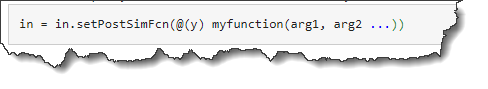This syntax is called an anonymous function. To learn more on those, I recommend going through this documentation page. This syntax allows you to pass inputs to the post-simulation function. For example, let's add two inputs to our previous function:
function newout = myPostSim3(out,bias,gain)
mySignal = out.yout.get('x').Values;
newout.meanWithBias = gain* (mean(mySignal) + bias);
end
With those additional inputs, you first create an anonymous function that will receive the Simulation Output object out as input, and pass it to myPostSim3 along with values for bias and gain:
mdl = 'massSpringDamper';
biasValue = 5;
gainValue = 10;
myAnonymousFcn = @(out) myPostSim3(out,biasValue,gainValue);
in = in.setPostSimFcn(myAnonymousFcn);
out = sim(in)
out =
Functionally, this one line of code defining myAnonymousFcn is equivalent to create a wrapper function around myPostSim3 that would look like this:
function newout = myNotAnonymousFcn(out)
biasValue = 5;
gainValue = 10;
newout = myPostSim3(out,biasValue,gainValue)
end
Here is an additional image that I hope will help differentiate the argument for which the value will be passed to the function when it will be called at the end of the simulation (Specified using @(argumentName) in front of the function signature), versus the arguments for which the value is passed immediately: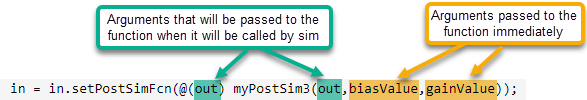## Specifying a method of an App or MATLAB Class as Callback Function

To illustrate this, I added two variables x0 and v0 specifying the initial position and velocity of the Second Order Integrator block.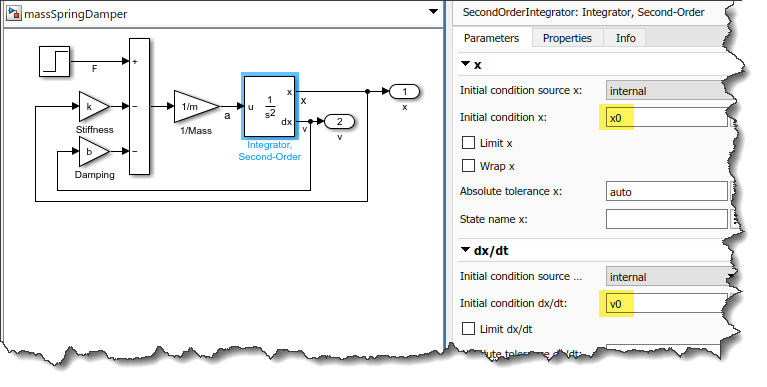To keep things simple, I will not use an App Designer app, but a simple class simulating the model multiple times with different initial values. The same principle would apply to an App developed in App Designer.
Here is what my simple class does:
• In the constructor, I store the name of the model to be simulated and use rng to initialize the random number generator
• In the runSim method, I define a random value x0 that will be used as the same initial position for all simulations
• I define different random values v0 to be used in each simulation
• I define the class method thePostSimFcn as post-simulation callback and I pass to it the values of x0 and v0 using additional arguments
• In the thePostSimFcn, I store x0 and v0 in the simulation output object for future use
classdef myClass
properties
mdl % the name of the model to simulate
end

methods
function obj = myClass(mdl)
rng('shuffle');
obj.mdl = mdl;
end

function out = runSim(obj,N)
x0 = rand; % Initialize all runs with same position
for i = 1:N
v0 = 10*rand; % Initialize runs with different velcity
in(i) = in(1).setVariable('x0',x0);
in(i) = in(1).setVariable('v0',v0);
in(i) = in(i).setPostSimFcn(@(out) obj.thePostSimFcn(out,x0,v0));
end
out = sim(in,'ShowProgress','off');
end

function newOut = thePostSimFcn(obj,out,x0,v0)
newOut = out;
% Store the ICs in the output for the record
newOut.x0 = x0;
newOut.v0 = v0;
end
end
end
Things to note here are:
• The first argument of the post-simulation function thePostSimFcn is obj, the object itself.
• When configuring the post-simulation callback, I refer to it as obj.thePostSimFcn because it is a method of this class.
I can then use my simple class with this code:
myObj = myClass('massSpringDamper');
out = myObj.runSim(2);
figure;
for i = 1:length(out)
subplot(2,1,1); hold on;
plot(out(i).yout.get('x').Values);
subplot(2,1,2); hold on;
plot(out(i).yout.get('v').Values);
legendTxt{i} = ['Run ' num2str(i) ' - x0=' num2str(out(i).x0) ' - v0=' num2str(out(i).v0)];
end
subplot(2,1,1); legend(legendTxt)